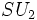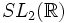# Book:Artin

View a complete list of books
View all terminology defined, facts proved, and facts stated in this book OR view all things referenced in this book
Algebra by Michael Artin, ISBN 0130047635, 13-digit ISBN 978-0130047632

### General information

Algebra is written by Michael Artin, Professor of Mathematics at Massachussetts Institute of Technology. It is an outgrowth of supplementary notes prepared by Artin for algebra classes he taught at MIT. According to Artin (Preface, written in December 1990), the main novel feature of the book is its increased emphasis on special topics. Artin enunciates three general guiding principles he tried to follow in his book:

1. The main examples should precede the abstract definitions
2. The book is not intended for a "service course", so technical points should be presented only if they are needed in the book.
3. All topics should be important for the average mathematician

Some topics that Artin's book lays more emphasis on than other books:

• The role of matrix groups
• Lattices, and groups acting on the plane and in Euclidean space

### Relevant contents in group theory

Chapter 2: Groups has the following subsections:

1. The definition of a group (pages 38-44; exercises on page 69) includes the following:
2. Subgroups (pages 44-48, exercises on pages 70-71) includes the following:
• Definition of subgroup
• Proof that every nontrivial subgroup of the group of integers is cyclic on its smallest element
• Definition of greatest common divisor of two integers
• Proof that the set of$n$ such that$x^n$ is the identity, is a subgroup of$\mathbb{Z}$
• Definition of order of a group
• Definition of cyclic group
• Definition of order of an element
• Definition of subgroup generated by a subset
• Example of the quaternion group as a non-cyclic small subgroup of$GL(2,\mathbb{C})$
3. Isomorphisms (pages 48-51, exercises on pages 71-72) includes the following:
4. Homomorphisms (pages 51-52, exercises on pages 72-73) includes the following:
5. Equivalence relations and partitions (pages 53-56, exercises on pages 73-74)
6. Cosets (pages 57-59, exercises on page 74) includes the following:
7. Restriction of a homomorphism to a subgroup (pages 59-61, exercises on pages 74-75):
8. Products of groups (pages 61-64, exercises on page 75)
9. Modular arithmetic (pages 64-66, exercises on page 76)
10. Quotient groups (ages 66-69, exercises on page 76)

Chapter 5: Symmetry has the following subsections:

1. Symmetry of plane figures
2. The group of motions of the plane
3. Finite groups of motions
4. Discrete groups of motions
5. Abstract symmetry: group operations
6. The operation on cosets
7. The counting formula
8. Permutation representations
9. Finite subgroups of the rotation group

Chapter 6: More group theory has the following subsections:

1. The operations of a group on itself
2. The class equation of the icosahedral group
3. Operations on subsets
4. The Sylow theorems
5. The groups of order 12
6. Computation in the symmetric group
7. The free group
8. Generators and relations
9. The Todd-Coxeter algorithm

Chapter 8: Linear groups has the following subsections:

1. The classical linear groups
2. The special unitary group$SU_2$
3. The orthogonal representations of$SU_2$
4. The special linear group$SL_2(\R)$
5. One-parameter subgroups
6. The Lie algebra
7. Translation in a group
8. Simple groups

Chapter 9: Group representations has the following subsections:

1. Definition of a group representation
2.$G$-invariant forms and unitary representations
3. Compact groups
4.$G$-invariant subspaces and irreducible representations
5. Characters
6. Permutation representations and the regular representation
7. The representations of the icosahedral group
8. One-dimensional representations
9. Schur's lemma, and proof of the orthogonality relations
10. Representations of the group$SU_2$

### Style of definitions

Most definitions are given in paragraphs, rather than under explicit definition headers. Usually the main symbols/equations used in the definition are labeled as a point.

As indicated by the guiding principle, definitions are typically introduced after some examples, motivation or general idea, and the definition may be buried within the example.

### Style of proofs

While there are some instances of a proposition followed by a proof, a large number of propositions are stated after the reasoning used in their proof is already given. Sometimes, full details from the proof are omitted, and clarification is left to the exercises. Some important (but easy) statements are left to the exercises.

### Style of exercises

Exercises are found at the end of each chapter, and are subdivided by sections in the chapter. There are also Miscellaneous problems at the end of the chapter. The exercises vary from general important facts to computations in specific examples.

## Use of the book

Artin's book is used as a textbook/reference book for advanced undergraduate algebra and is also recommended as background reading for certain graduate programs/qualifying examinations in some universities. PLACEHOLDER FOR INFORMATION TO BE FILLED IN: [SHOW MORE]

## Comparison with other books

• Book:DummitFoote: The book by Dummit and Foote has clearer definitions, a more rigorous presentation, and focuses more on finite groups and permutation groups as opposed to matrix groups.
• Book:Herstein